Thermochemistry (2016 VCE) 1)A senior Chemistry student bought a packet of Krispy Krackers from the local farmers’ market. The label on the packet had no information on the energy content of the biscuits. The student decided that he would measure the energy content of a Krispy Krackers biscuit by burning it under a can of water and measuring the temperature rise of the water. Having performed a similar experiment in class, he knew that when the biscuit was burnt, heat energy would be lost to the environment. To increase the accuracy of the result, some butane gas from a butane canister was first burnt and the temperature rise of the water from that was measured. The heat energy released by burning butane was known, and the percentage energy loss using the equipment could be determined and adjusted to get the result for the biscuit. The experimental set-up and the results for Part 1 of the experiment are shown below.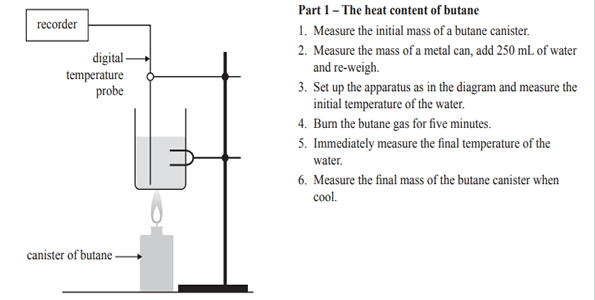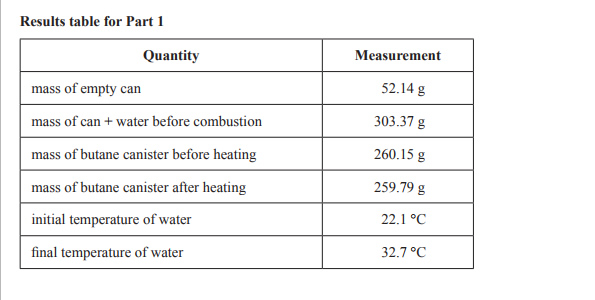a) Write the balanced thermochemical equation for the complete combustion of butane Solutionb) Calculate the amount of heat energy absorbed by the water when it was heated by burning the butane. Give your answer in kilojoules.   Solutionc) Calculate the experimental value of the molar heat of combustion of butane. Give your answer in kJ mol–1.   Solutiond) Use the known enthalpy change for butane to calculate the percentage energy loss to the environment using the following relationship.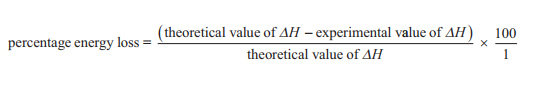Solutione) The experimental set-up and the results for Part 2 of the experiment are shown below.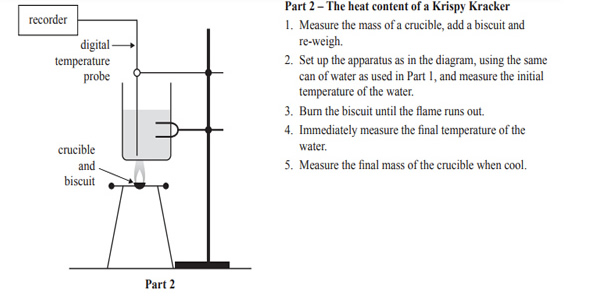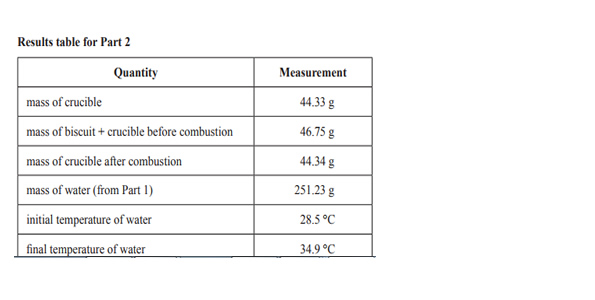Calculate the energy content of Krispy Krackers using the data in the results table for Part 2. Give your answer in kJ/100 g. Solution f) Explain why the energy content of a biscuit cannot be given in kJ mol–1. Solutiong) Assume that the same percentage heat energy loss occurred when burning the Krispy Kracker as when the butane was burnt in Part 1. Calculate a more accurate value of the energy content of Krispy Krackers in kJ/100 g Solution2) Methanol is a liquid fuel that is often used in racing cars. The thermochemical equation for its complete combustion is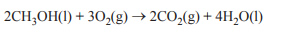Octane is a principal constituent of petrol, which is used in many motor vehicles. The thermochemical equation for the complete combustion of octane is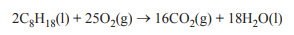The molar mass of methanol is 32 g mol–1 and the molar mass of octane is 114 g mol–1. Which one of the following statements is the most correct? A. Burning just 1.0 g of octane releases almost 96 kJ of heat energy. B. Burning just 1.0 g of methanol releases almost 23 kJ of heat energy. C. Octane releases almost eight times more energy per kilogram than methanol. D. The heat energy released by methanol will not be affected if the oxygen supply is limited. Solution3) The combustion of hexane takes place according to the equation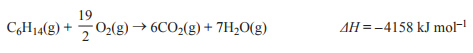Consider the following reaction.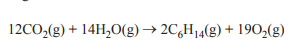The value of ΔH, in kJ mol–1, for this reaction is A. +8316 B. +4158 C. –2079 D. –3568 Solution4) A chemical reaction has the following energy profile.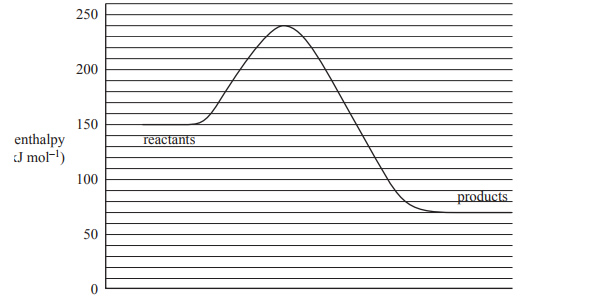The enthalpy change of the forward reaction, in kJ mol–1, is A. –170 B. –80 C. +70 D. +240 Solution5) A student calibrated a calorimeter using an electric heating coil. A current of 1.50 A with a potential difference of 4.50 V was applied for two-and-a-half minutes. A digital probe recorded a temperature rise of 5.35 °C. The value of the calibration factor, in J °Cl–1, is A. 189 B. 42.1 C. 3.15 D. 0.317 Solution